# PHY3: TEMPERATURE

##### This unit is about temperature and also talks about the gas laws.

TEMPERATURE

Temperature describes the extent to which heat is concentrated in an object.  It is defined as the measure of the degree of coldness or hotness of an object.

NB

The total energy in a body depends more on the mass of the object than the temperature of the object

Example

The amount of energy in a basin full of water at 40oc is more than that in a mug or cup full of water at 90oc

Temperature scales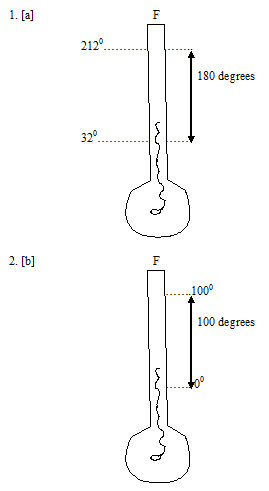1. [a] The Fahrenheit scale

This scale uses 3parts. It is one of the earliest scales used but only used in U.S of late. It uses O0F for the freezing point of an ice and salt mixture tt 320F for the freezing point of pure water and 98oF for the normal temperature of the human body.

The temperature using this thermometer depends on the rise or fall of the liquid length inside a thermometer when temp rises or falls. These are called liquid – in – glass thermometers.

1. [b] Celsius scale

This scale uses two fixed points. These are;-

The lower fixed point [lfp]

This is the temp of pure melting ice and is taken to be O0c. Impurities in ice would lower its melting point.

Upper fixed point [ufp]

The ufp is the temperature of the stream above water boiling at normal atmospheric pressure of 760 mm ttg and is taken to be 100oc.

Note

Temperature of the boiling point of water is not used because any impurities in water will raise its boiling point while the temperature of the stream is not affected by impurities. The following formulae can be used to convert OF to oC scale or vice versa.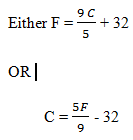Methods of finding fixed point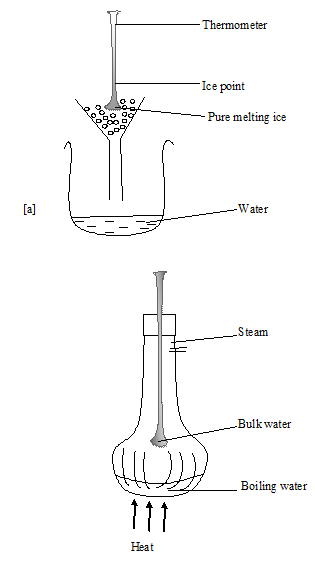Cont.

The level of mercury in the thermometer when placed in pure melting ice and in stream above boiling water are marked by using a scratches. The level in [a] shows the lower fixed point while that in [b] is the upper fixed point.

The distance between the marked levels is then calibrated or divided into 100 equal parts thus each division will be equal to 1oc

Example

A student found out that the distance between the lower and the upper fixed points on her designer thermometer is 15cm. If she divides this interval into 100 equal parts what is the temp in oc if the reading of the mercury is 5cm above the lower fixed point when the thermometer is placed on another body.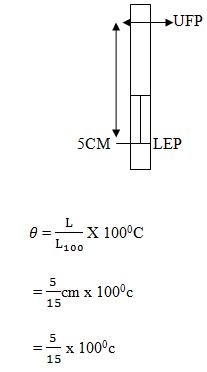= 33.3oc

BRIEF HISTORY ABOUT TEMPERATURE

Common liquids used in thermometers

Mercury

This is a liquid metal which expands significantly when exposed to heat. If the bore of the tube in which this mercury is kept is constant then the volume that expands is proportional to the length.

A very sensitive thermometer must therefore have a very small or narrow bore of the tube. Mercury is opaque hence it can be easily seen. It has a high boiling point of 6300c. However, mercury freezes at a temperature of 410c thus providing a good range 4 temp measurement.

Mercury vapour is, however, poisonous and mercury frizzes at slightly a high temperature of – 41oc. Hence such a thermometer is better for measuring temperatures of better bodies.

Alcohol [ethanol]

Alcohol has one advantage over mercury it has a very low freezing point of – 1150c however, alcohol boils at only 780c. Hence alcohol – in – glass thermometers are better for taking measurements in cooler regions.

Alcohol is not easily seen because it’s clear but can be coloured to make it visible. Alcohol wets the glass tube thus the readings taken may not be accurate.

Water

Water cannot be used thermometric liquid because;-

1. Its freezing point of o0c is too high
2. It has an irregular expansion
• It is clear and wets the tube.
• Kelvin scale [thermodynamic scale]

This scale uses one fixed point called the absolute zero [ok]

The absolute zero is the lowest possible temperature at which all the energy available in a body has been removed. This is the most common scale used and it is the S.I unit for measurement of temperature. The absolute zero is equal to 273.150c

Ok = 273.150c

But the commonly used value of absolute zero ok is -273oC ice point of water is therefore;-

[0oc]=273k

The boiling point of water which is 100oc is 373k. Each Kelvin [K] is, therefore equal to 10c

T is the symbol used for temperature on the Kelvin scale [thermomal dynamic scale which O is the symbol used 2 represent temperatures on the calcium scale. The following formulae can be used to convert the Kelvin scale to calcium scale or vice versa.

T = [ 0 + 273] k

Or

0  [J – 273] 0C

THE GAS LAWS

The gas laws are concerned with the behaviors of gases in relation to 3 varying quantities;-

Volume

Pressure

Temperature [T] for a fixed mass of gas to study how the changes in one quality affect the other. The 3rd quantity must be kept constant.

Boyle’s law [t]

Boyle investigated the relationship between variations of pressure between a gas with its volume when the temperature of the system is kept constant.

Boyle’s low, therefore, states that the volume of a fixed mass of gas is inversely proportional to the pressure provided the temperature remains constant.

An experiment to investigate Boyle’s lowfer gases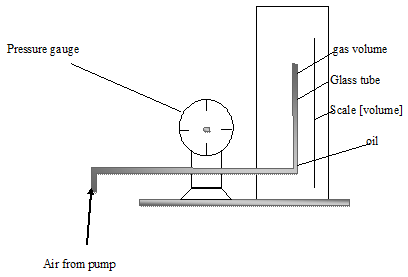Pressure from the pump is measured from the pressure gauge while the corresponding variation of volume is read from the volume scale. A graph of pressure plotted against volume is a smooth curve as shown below.

A graph of pressure against volume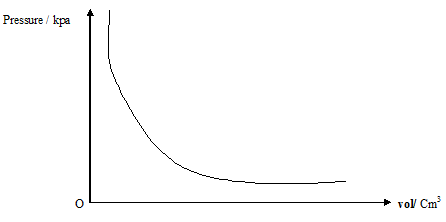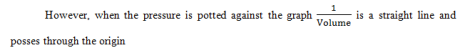This graph shows that pressure is directly proportional to the reciprocal of the volume i.e. pressure is inversely proportional to the volume.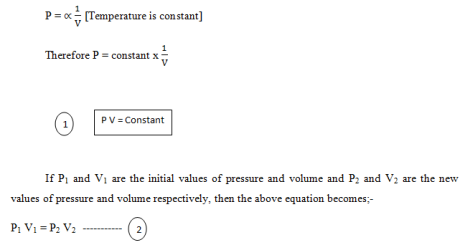Note

Although air was used to carry this investigation Boyle’s law is true for all gases over a wide range of pressures.

Example

If a certain quantity of gas has a volume of 30cc and a pressure of 100,000 pa. What is its volume when the pressure is 500pa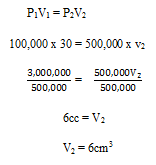Charles’ law

Charles’ law involves the relationship between volume and the absolute temperature of a fixed mass of gas at constant pressure is directly proportional to its absolute temperature.

The following apparatus is used to investigate Charles’ law for gases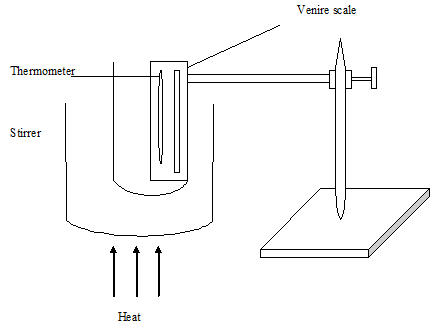During the investigation, the atmospheric pressure is kept constant. This is done by adjusting the mercury thread level such that every time the reading is taken the level is at the same position.

The corresponding temperature is recorded together with the volume and these are read from the thermometer and the volume scale respectively

A graph of volume against absolute temperature is then plotted and a straight line that cuts the volume axis at an intercept is obtained.

When this graph is extrapolated [extended] backward, it meets the obsolete temperature axis at absolute zero, [ok] i.e. at 273oc.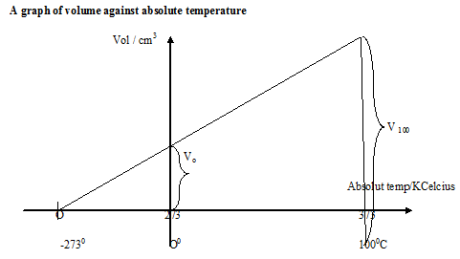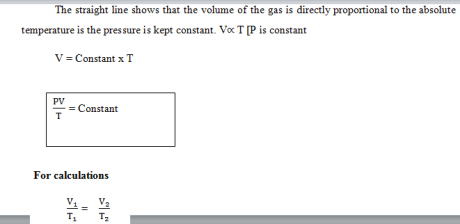Using the kinetic theory of gases, the rise in temperature causes the particles of the gas to travel faster and hence exert a larger force on the wall of the container.

If the pressure remains constant, then the volume must increase. For Boylle’s law a reduction volume without change in temp of system that the particles become closer together to collide with the wall of the container more frequently leading to an increase in pressure

A gas of volume 2cc at 270c is heated to 3270c. What is its new volume if the pressure is constant?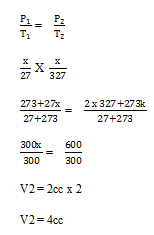The pressure and law[V]

The law explains the behavior of a fixed mass of gas at constant volume when the pressure changes with absolute temperature. Pressure law states that the pressure of a fixed mass of gas at constant volume directly proportional to its absolute temperature.

The following apparatus may be used to investigate the pressure law.During the experiment the volume of air in the flask is kept constant as the temp of water is changed by heating, the corresponding change in pressure can be monitored and recorded from the pressure gauge.

A graph of pressure against absolute is then platted and a straight line that cuts his pressure axis at an intercept is obtained. If this line is further extrapolated it will cut the absolute temperature axis at absolute zero [10k

A graph of pressure against absolute temperature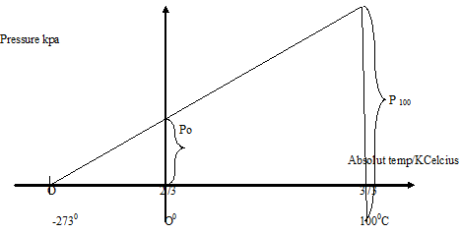The straight line shows that pressure varies/ directly proportional to the absolute temperature provided the volume is constant.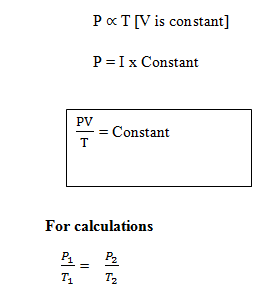The gas equation [equation of state]

The gas equation represents the summary of three equations of Boyle’s Charles and pressure laws i.e;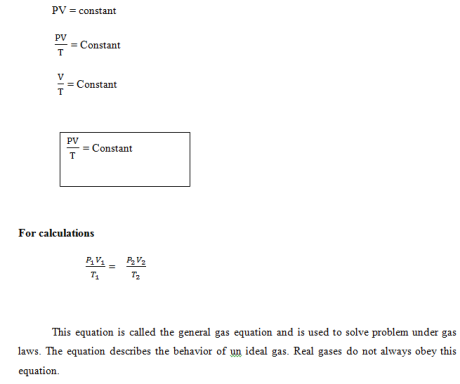THIS VIDEO SHOWS EXPERIMENTS ABOUT GAS LAWS

The standard temperature and pressure [s.t.p]

The s.t.p represents the volume a gas will occupy at a temperature of Ooc and 760mmtty thus 760mmtty and O0 are called s.t.p

Examples

Cycle/ bicycle pump contain 50cm3 of air at 170c and pressure of 1.0 atmosphere. Find the pressure when the air is compressed to 10cm3 and its temperature rises to 270c

P1 = 1.0 atmosphere

1. A volume of 2,500cm3 of hydrogen gas is collected at 670c at a pressure of 730 mmttg. Calculate the volume of the gas at s.t.p.

V1 = 2500cm3

P1 = 730mmttg

T1 = 67oc

= 67 + 223

= 340k

V2 =?

P2 = 760

T2 =Ooc

= 273 + O

= 273k## STEM Elearning

We at FAWE have built this platform to aid learners, trainers and mentors get practical help with content, an interactive platform and tools to power their teaching and learning of STEM subjects, more

#### How to find your voice as a woman in Africa

© FAWE, Powered by: Yaaka DN.